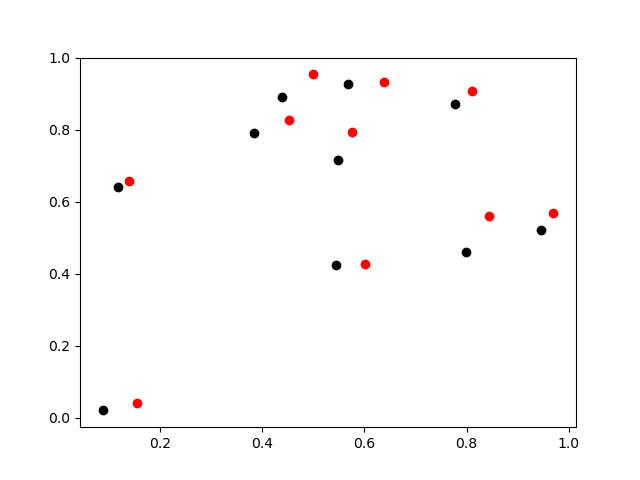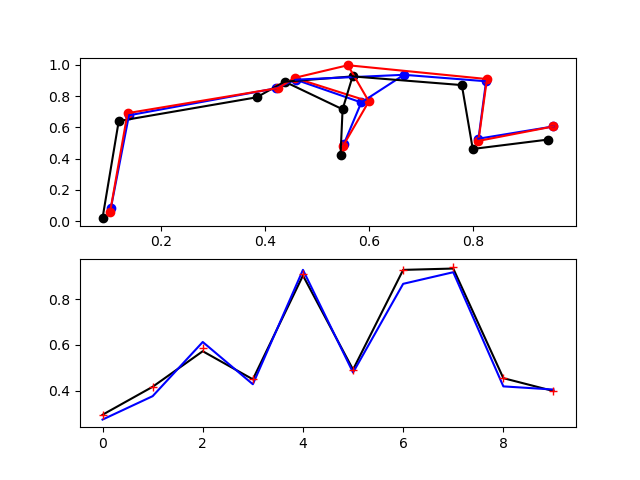# 初始化坐标参数

# constrain.py
import numpy as np
import matplotlib.pyplot as plt

np.random.seed(0)
N = 10
crd = np.random.random((N, 3))

plt.figure()
plt.plot(crd[:,0], crd[:,1], 'o', color='black')
plt.savefig('initial.png')# 坐标的更新

# constrain.py
import numpy as np
import matplotlib.pyplot as plt

np.random.seed(0)
N = 10
crd = np.random.random((N, 3))
dt = 0.1
vel = np.random.random((N, 3))
new_crd = crd + vel * dt

plt.figure()
plt.plot(crd[:,0], crd[:,1], 'o', color='black')
plt.plot(new_crd[:,0], new_crd[:,1], 'o', color='red')
plt.savefig('move.png')# 定义成键关系

# constrain.py
import numpy as np
import matplotlib.pyplot as plt

np.random.seed(0)
N = 10
crd = np.random.random((N, 3))
dt = 0.1
vel = np.random.random((N, 3))
new_crd = crd + vel * dt

bonds = np.array([[0,1],[0,2],[0,4],[2,3],
[2,4],[3,8],[5,8],[4,6],
[6,7],[7,9]])

plt.figure()
plt.plot(crd[:,0], crd[:,1], 'o', color='black')
plt.plot(new_crd[:,0], new_crd[:,1], 'o', color='red')
for bond in bonds:
plt.plot(crd[bond][:,0], crd[bond][:,1], color='green')
plt.plot(new_crd[bond][:, 0], new_crd[bond][:, 1], color='purple')
plt.savefig('move.png')# LINCS算法# constrain.py
import numpy as np
from jax import numpy as jnp
from jax import grad, jit, vmap
import matplotlib.pyplot as plt

# Initialization
np.random.seed(0)
N = 10
Dimension = 3
crd = np.random.random((N, Dimension))
# Mass diag
M = np.random.random(N)
Mi = np.identity(N) * M
Mii = np.identity(N) * (M ** (-1))
dt = 0.1
vel = np.random.random((N, Dimension))
new_crd = crd + vel * dt

bonds = np.array([[0,1],[0,2],[0,4],[2,3],
[2,4],[3,8],[5,8],[4,6],
[6,7],[7,9]])
# Bond length
di = np.linalg.norm(crd[bonds[:,0]] - crd[bonds[:,1]], axis=1)

# Automatic differentiation
def B(new_crd, bond, crd):
return jnp.linalg.norm(new_crd[bond]-new_crd[bond]) -\
jnp.linalg.norm(crd[bond]-crd[bond])
B_value = B_vmap(new_crd, bonds, crd)

# LINCS
ccrd = new_crd.copy()
tmp0 = jnp.einsum('ij,kjl->kil', Mii, B_value)
tmp1 = jnp.einsum('jil,kil->jk', B_value, tmp0)
tmp2 = np.linalg.inv(tmp1)
tmp3 = jnp.einsum('ijk,jk->i', B_value, new_crd)-di
tmp4 = jnp.einsum('ij,j->i', tmp2, tmp3)
tmp5 = jnp.einsum('ijk,i->jk', B_value, tmp4)
tmp6 = jnp.einsum('ij,jk->ik', Mii, tmp5)
ccrd -= tmp6

# Draw
plt.subplot(211)
plt.plot(crd[:,0], crd[:,1], 'o', color='black')
plt.plot(new_crd[:,0], new_crd[:,1], 'o', color='blue')
plt.plot(ccrd[:,0], ccrd[:,1], 'o', color='red')
for bond in bonds:
plt.plot(crd[bond][:,0], crd[bond][:,1], color='black')
plt.plot(new_crd[bond][:,0], new_crd[bond][:,1], color='blue')
plt.plot(ccrd[bond][:, 0], ccrd[bond][:, 1], color='red')

plt.subplot(212)
di = np.linalg.norm(crd[bonds[:,0]] - crd[bonds[:,1]], axis=1)
diuc = np.linalg.norm(new_crd[bonds[:,0]] - new_crd[bonds[:,1]], axis=1)
dic = np.linalg.norm(ccrd[bonds[:,0]] - ccrd[bonds[:,1]], axis=1)
plt.plot(di, color='black')
plt.plot(diuc, color='blue')
plt.plot(dic, '+', color='red')
plt.savefig('move.png')# LINCS算法原理以及代码实现思路

$\frac{d^2\textbf r}{dt^2}=\textbf M^{-1}\textbf f$

LINCS约束的方程可以表述为K个方程：

$g_i(\textbf r)=|\textbf r_{i1}-\textbf r_{i2}|-d_i=0\ \ \ \ i=1,...,K$

$-\textbf M\frac{d^2\textbf r}{dt^2}=\frac{\partial}{\partial\textbf r}(\textbf V-\lambda \cdot g)$

$B_i=\frac{\partial g_i}{\partial r_i}$

$\textbf r_{n+1}=\textbf r_{n+1}^{unc}-\textbf M^{-1}B_n(B_n\textbf M^{-1}B_n^T)^{-1}(B_n\textbf r_{n+1}^{unc}-\textbf d)$

$v_{n+\frac{1}{2}}=\frac{r_{n+1}-r_n}{\Delta t}$

## 注意事项一

$$\textbf r_{n+1}$$是基于$$\textbf r_{n+1}^{unc}$$来进行调整的，但是如果一开始直接使用：

r=r_unc


# 参考链接

posted @ 2022-02-09 21:41  DECHIN  阅读(355)  评论(1编辑  收藏  举报